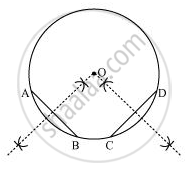# Suppose You Are Given a Circle. Give a Construction to Find Its Centre. - Mathematics

Suppose you are given a circle. Give a construction to find its centre.

#### Solution

The below given steps will be followed to find the centre of the given circle.

Step1. Take the given circle.

Step2. Take any two different chords AB and CD of this circle and draw perpendicular bisectors of these chords.

Step3. Let these perpendicular bisectors meet at point O. Hence, O is the centre of the given circle.Concept: Circles Passing Through One, Two, Three Points
Is there an error in this question or solution?
Chapter 10: Circles - Exercise 10.3 [Page 176]

#### APPEARS IN

NCERT Class 9 Maths
Chapter 10 Circles
Exercise 10.3 | Q 2 | Page 176

Share